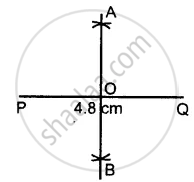# Draw a Line Segment Pq = 4.8 Cm. Construct the Perpendicular Bisector of Pq. - Mathematics

Sum

Draw a line segment PQ = 4.8 cm. Construct the perpendicular bisector of PQ.

#### Solution

Steps of Construction :1. Draw a line segment PQ = 4.8 cm.
2. With P as centre and radius equal than half of PQ, draw an arc on both the PQ.
3. With Q as the centre and the same radius as taken in step 2, draw arcs on both sides of PQ.
4. Let the arcs intersect each other at point A and B
5. Join A and B.
6. The line AB cuts the line segment PQ at the point O. Here OP = OQ and ∠AOQ = 90°. Then the line AB is a perpendicular bisector of PQ.
Is there an error in this question or solution?
Chapter 18: Constructions - Exercise 18 (B) [Page 204]

#### APPEARS IN

Selina Concise Mathematics Class 8 ICSE
Chapter 18 Constructions
Exercise 18 (B) | Q 2 | Page 204
Share